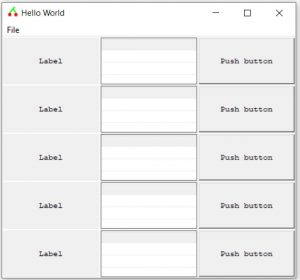# SOB – Container – Matrix

A matrix (I suppose you could also think of it as a grid) imposes on to its child:

Positioning:

• In a uniform row/column matrix

Dimensions:

• All child objects have the height of the tallest child object, and the width of the widest child object.

A Matrix definition requires two special parameters:

• Row or Column – a matrix must have 1 defined dimension, either the number of rows, or the number of columns
• Dimension – an integer

So to define a matrix as having 3 columns we might use something like:

`newscript`
`dim myWindow = sob (0, "add","window","Hello World")`
`function ClickMyWindow ( sobid )`
`sob ( myWindow, "delete")`
`quit`
`End function`
`sob ( myWindow , "ON" , "CLICK" , "ClickMyWindow" )`
`dim myMenuBar = sob (myWindow , "add" , "menu" , "bar" )`
`dim myMenuFile = sob (myMenuBar , "add" , "menu" , "Horizontal" , "File" )`
`dim myFileExit = sob ( myMenuFile , "add" , "menu" , "Vertical" , "Exit" )`
`sob ( myFileExit , "ON" , "CLICK" , "ClickMyWindow")`

`dim myContainer = sob ( myWindow , "add" , "CONTAINER" , "MATRIX.C" , 3)`
`sob ( myContainer, "add" ,"label" , "COLUMN" )`
`sob ( myContainer, "add" , "LISTVIEW"," ", 5,0x00800008 )`
`sob ( myContainer, "add" , "button" , "Push" , "Push button" )`

`sob ( myContainer, "add" ,"label" , "Label" )`
`sob ( myContainer, "add" , "LISTVIEW"," ", 5,0x00800008 )`
`sob ( myContainer, "add" , "button" , "Push" , "Push button" )`

`sob ( myContainer, "add" ,"label" , "Label" )`
`sob ( myContainer, "add" , "LISTVIEW"," ", 5,0x00800008 )`
`sob ( myContainer, "add" , "button" , "Push" , "Push button" )`

`sob ( myContainer, "add" ,"label" , "Label" )`
`sob ( myContainer, "add" , "LISTVIEW"," ", 5,0x00800008 )`
`sob ( myContainer, "add" , "button" , "Push" , "Push button" )`

`sob ( myContainer, "add" ,"label" , "Label" )`
`sob ( myContainer, "add" , "LISTVIEW"," ", 5,0x00800008 )`
`sob ( myContainer, "add" , "button" , "Push" , "Push button" )`

`sob ( myWindow, "SET" , "SHRINK" )`

Which would produce a GUI as shown in the following screenshot:Or if we changed the matrix definition line to:

`dim myContainer = sob ( myWindow , "add" , "CONTAINER" , "MATRIX.R" , 3)`

We would see:##### Functional specifications for matrix container related SOB commands:
`SOB ( _`
`<Parent: number expression> ,_`
`"ADD" , _"CONTAINER" , _`
`"container type" , _``<FIXED: integer expression>  _`
`)`
Where `container type` (enclosed in a pair of `"` ) must be replaced by one of the following (case insensitive):
And the `<FIXED: integer expression> `defines the number of columns / rows as appropriate.